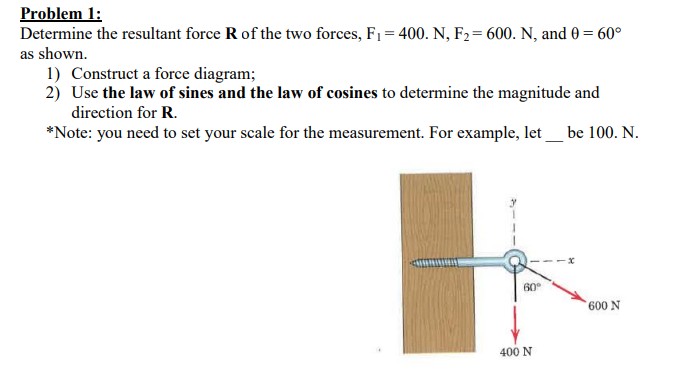# Problem 1Determine the resultant force R of the two forces, F= 400. N, F2= 600. N, and 0 60°as shown1) Construct a force diagram;2) Use the law of sines and the law of cosines to determine the magnitude anddirection for R*Note: you need to set your scale for the measurement. For example, let be 100. N.60600 N400 N

Question
29 viewshelp_outlineImage TranscriptioncloseProblem 1 Determine the resultant force R of the two forces, F= 400. N, F2= 600. N, and 0 60° as shown 1) Construct a force diagram; 2) Use the law of sines and the law of cosines to determine the magnitude and direction for R *Note: you need to set your scale for the measurement. For example, let be 100. N. 60 600 N 400 N fullscreen
check_circle

Step 1

Hi there! Since there are multiple questions we will answer the first question for you. If you need help with the other parts kindly post  them separately.

Step 2

The resultant of the forces can be calculated by using the cosine rule, which is given as,

Step 3

The given values are F1 = 400 N, F2 = 600 N and θ = 60o

Substituting...

### Want to see the full answer?

See Solution

#### Want to see this answer and more?

Solutions are written by subject experts who are available 24/7. Questions are typically answered within 1 hour.*

See Solution
*Response times may vary by subject and question.
Tagged in

### Solid Mechanics